# Damped Oscillators

An oscillator is anything that has a rythmic periodic response. A damped oscillator has a response that fades away over time. Examples include a swinging pendulum, a bobbing weight on a spring, and also a resistor - inductor - capacitor (RLC) circuit.

The interactive RLC simulation is nice, but what equations drive it? Is there a better approach than a numerical simulation? What component values make it under-damped? Over-damped? When is it a critically-damped oscillator?

Mathemeticians have completely solved the equations that govern a damped oscillator. My web page shows their results, and explains how to compute frequency and critically-damped parts values.

## EquationsSuppose you have an RLC circuit, which has a resistor + inductor + capacitor in series. When the switch closes at time t=0 the capacitor will discharge into a series resistor and inductor.

What is the voltage V and current I as a function of time?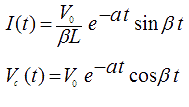where: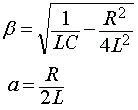and V= initial voltage
R = resistance (ohms)
L = inductance (henrys)
e = base of natural log (2.71828...)

## What Does This Mean?

The above equation is the current for a damped sine wave. It represents a sine wave of maximum amplitude (V/BL) multiplied by a damping factor of an exponential decay. The resulting time variation is an oscillation bounded by a decaying envelope.

## Critical Damping

We can use these equations to discover when the energy dies out smoothly (over-damped) or rings (under-damped).

Look at the term under the square root sign, which can be simplified to: R2C2-4LC

 When R2C2-4LC is positive, then α and β are real numbers and the oscillator is over-damped. The circuit does not show oscillation.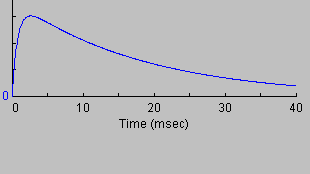When R2C2-4LC is negative, then α and β are imaginary numbers and the oscillations are under-damped. The circuit responds with a sine wave in an exponential decay envelope.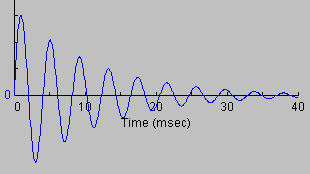When R2C2-4LC is zero, then α and β are zero and oscillations are critically damped. The circuit response shows a narrow peak followed by an exponential decay.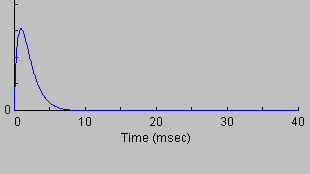## Resonant Frequency

The equations above will tell us the value as a function of time, but what we really want to know is the frequency of oscillation.

An LC circuit will oscillate at an angular frequency of: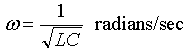To convert radians/sec to frequency f in Hertz, simply divide by 2p to get this: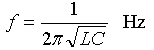To convert Hz to time period T, use the reciprocal of frequency: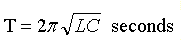## Examples

Question: What is a coilgun's firing period if it has an RLC circuit where R=0 and C=10,000 µF and L=100 µH?

Answer: The firing period is only the first half-wave of the oscillation.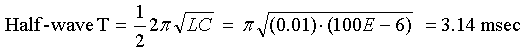Question: What resistance will make this coilgun critically damped?

Answer: Solve R2C2=4LC for resistance R to get: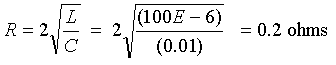< Previous Page 5 of 6 Next >